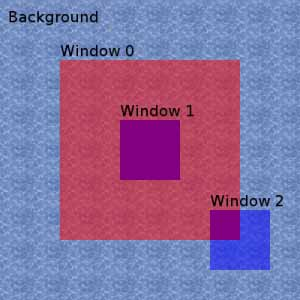### Problem Statement

You are using an operating system in which each application opens in a rectangular window with some level of transparency. The desktop, which lies beneath all the windows, shows a picture. A pixel in that picture is clearly visible if there are N or less windows above it.

The screen is 100 x 100 pixels. You are given int[]s x1, y1, x2 and y2. The top-left corner of the ith window is at (x1[i], y1[i]), and the bottom-right corner is at (x2[i], y2[i]). Each window covers the rectangular area between its two corner coordinates, inclusive. For example, the window from (1, 1) to (2, 2) covers exactly four pixels. Return the total number of pixels that are not visible in the desktop picture.

### Definition

 Class: FancyGUI Method: totalDarkArea Parameters: int, int[], int[], int[], int[] Returns: int Method signature: int totalDarkArea(int N, int[] x1, int[] y1, int[] x2, int[] y2) (be sure your method is public)

### Constraints

-N will be between 0 and 50, inclusive.
-x1, y1, x2 and y2 will each contain between 0 and 50 elements, inclusive.
-x1, y1, x2 and y2 will contain the same number of elements.
-Each element of x1, y1, x2 and y2 will be between 1 and 100, inclusive.
-The ith element of x2 will be greater than or equal to the ith element of x1.
-The ith element of y2 will be greater than or equal to the ith element of y1.

### Examples

0)

 `1` `{21, 41, 71}` `{21, 41, 71}` `{80, 60, 90}` `{80, 60, 90}`
`Returns: 500`
 The purple colored area is the area of the desktop that you can't see. It is the result of the intersection between the 0th and 1st windows and the intersection of the 0th and 2nd windows. Each of the other desktop pixels is covered by at most one window.1)

 `2` `{1, 21, 41}` `{1, 21, 41}` `{100, 80, 60}` `{100, 80, 60}`
`Returns: 400`
2)

 `0` `{10}` `{20}` `{19}` `{29}`
`Returns: 100`
 Any window obscures the background picture.
3)

 `1` `{}` `{}` `{}` `{}`
`Returns: 0`
 There can be no windows at all, and N can be greater than the number of windows.

#### Problem url:

http://www.topcoder.com/stat?c=problem_statement&pm=7888

#### Problem stats url:

http://www.topcoder.com/tc?module=ProblemDetail&rd=10790&pm=7888

mohamedafattah

#### Testers:

PabloGilberto , brett1479 , Olexiy , ivan_metelsky

#### Problem categories:

Brute Force, Geometry, Simple Search, Iteration